• 本示例说明了如何应用imfilter函数，使用包含相等权重的5×5滤镜（通常称为平均滤镜）对2D灰度图像进行滤波。该示例还显示了如何使用相同的滤镜对真彩色（RGB）图像进行滤波。真彩色图像是大小为[m,n,3]的矩阵，其中...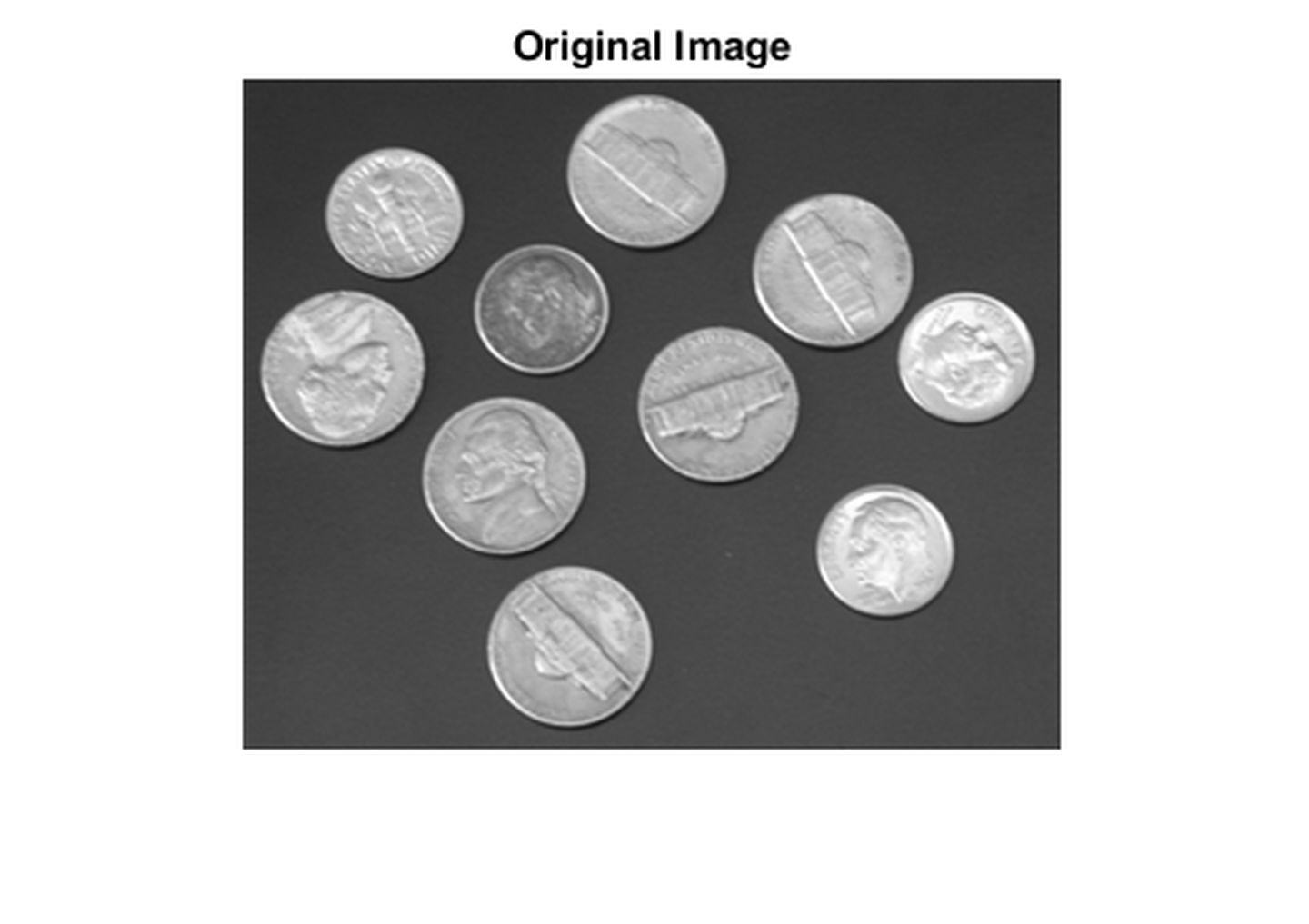本示例说明了如何应用imfilter函数，使用包含相等权重的5×5滤镜（通常称为平均滤镜）对2D灰度图像进行滤波。该示例还显示了如何使用相同的滤镜对真彩色（RGB）图像进行滤波。真彩色图像是大小为[m,n,3]的矩阵，其中最后一维表示三个颜色通道。使用2-D滤镜滤波真彩色图像等效于使用相同的2-D滤镜分别滤波图像的每个平面。有几种执行2D和多维滤波的MATLAB®函数可以与imfilter进行比较。函数filter2执行二维相关，conv2执行二维卷积，convn执行多维卷积。但是，这些滤波功能中的每一个始终将输入转换为double，而输出始终为double。同样，这些MATLAB®滤波函数始终假定输入为零填充，并且不支持其他填充选项。相反，imfilter不会将输入图像转换为double。该imfilter功能还提供了一组灵活的边界填充选项。使用均值滤波器滤波二维灰度图像将灰度图像读取到工作区中。 I = imread('coins.png');显示原始图像。 figure
imshow(I)
title('Original Image')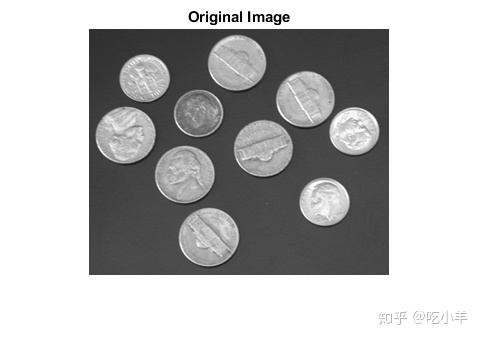创建一个标准化的5×5均值滤波器。 h = ones(5,5)/25;使用imfilter，将均值滤波器应用于灰度图像。 I2 = imfilter(I,h);显示滤波后的图像。 figure
imshow(I2)
title('Filtered Image')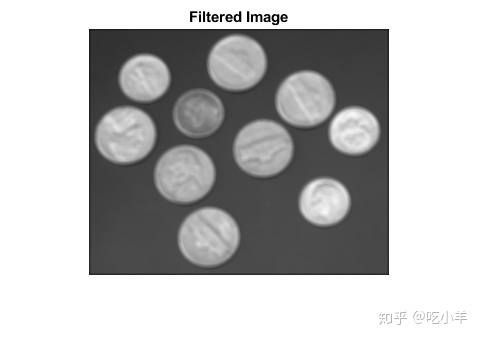使用imfilter滤波多维真彩（RGB）图像将真彩色图像读入工作区。 rgb = imread('peppers.png');
imshow(rgb);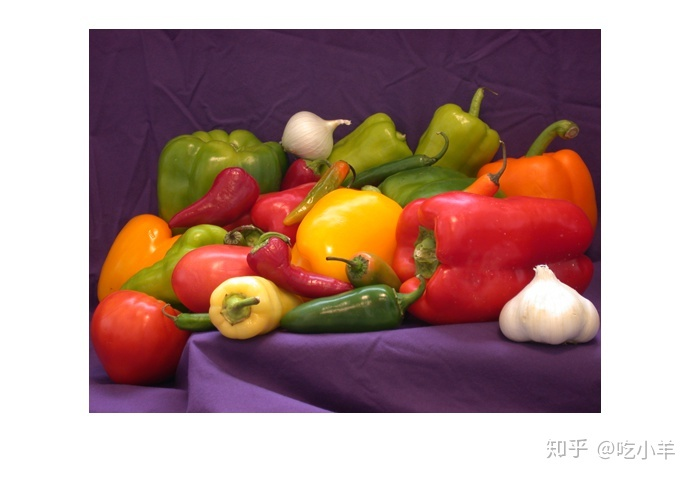创建一个滤波器。该均值滤波器包含相等的权重，并使滤波的图像看起来比原始图像更加模糊。 h = ones(5,5)/25;使用imfilter滤波图像并显示。 rgb2 = imfilter(rgb,h);
figure
imshow(rgb2)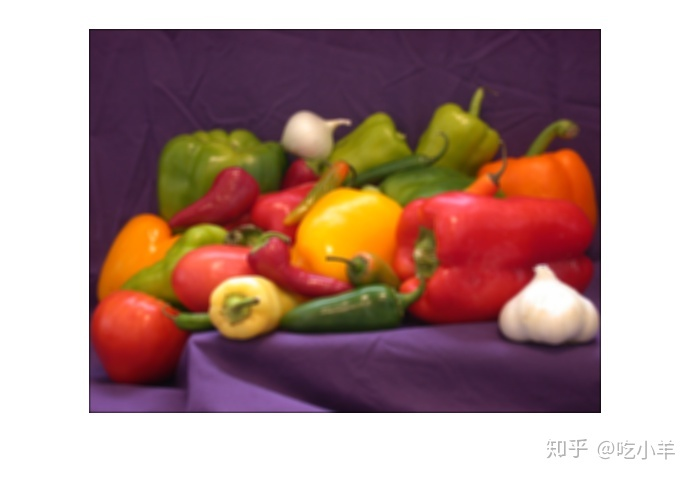注：本文根据MATLAB官网内容修改而成。我推荐给你以下的免费视频教程，特点是没有PPT，不掺水，直接编程环境下的实操课程：用100分钟了解MATLAB编程《MATLAB编程》视频课程 - 商业数据分析_数据可视化_Tableau免费课程_Tableau培训_自学Tableau_上海_1data.pro​www.1data.pro欢迎您进一步了解以下MATLAB系列文章：吃小羊：MATLAB作图实例：00：索引​zhuanlan.zhihu.com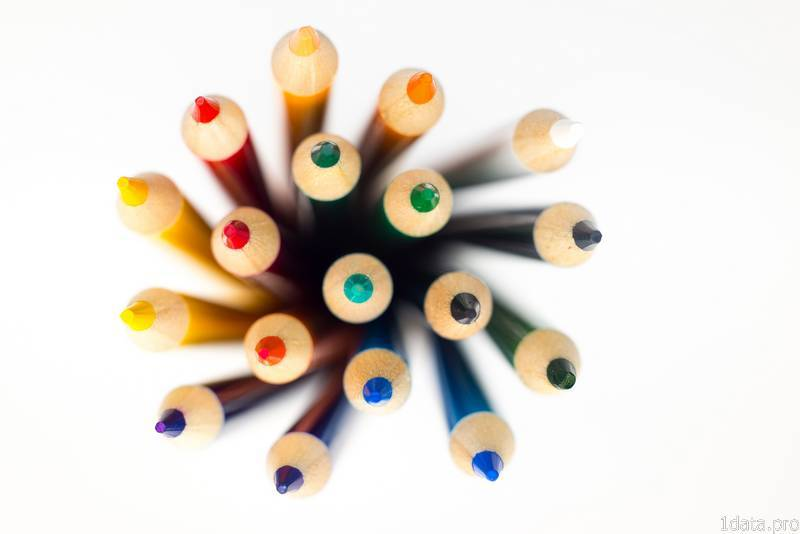吃小羊：MATLAB金融工具箱：00：索引​zhuanlan.zhihu.com展开全文• ## matlabRGB图转灰度图

千次阅读 2014-07-05 14:19:20
imgrgb = imread('flower.jpg'); imwrite(imgrgb, 'flower.bmp', 'bmp'); % jpg格式转换为bmp格式 imggray = rgb2gray(imgrgb);...imwrite(imggray, 'flower_grayscale.bmp', 'bmp'); % 存储为灰度图像


imwrite(imgrgb, 'flower.bmp', 'bmp'); % jpg格式转换为bmp格式

imggray = rgb2gray(imgrgb);

imwrite(imggray, 'flower_grayscale.bmp', 'bmp'); % 存储为灰度图像

展开全文• (一)：彩色图像转灰度图1、设计任务1) 读入彩色和灰度图像并显示；2) 对彩色图像转化为灰度图像并显示；3) 比较两种方法的效果。2、设计目的1) 掌握彩色图转灰度图的基本原理与方法；2) 初步掌握MATLAB的使用方法；3...
(一)：彩色图像转灰度图1、设计任务1) 读入彩色和灰度图像并显示；2) 对彩色图像转化为灰度图像并显示；3) 比较两种方法的效果。2、设计目的1) 掌握彩色图转灰度图的基本原理与方法；2) 初步掌握MATLAB的使用方法；3) 了解MATLAB在数字信号处理，尤其是图像处理中显现出来的优势。3、源代码% 把RGB格式的图片转换为YUV格式。clear; clc;x=imread('lena512.BMP');[line,row,dim]=size(x);x1=double(x); % 数据类型转换subplot(1,3,1) % 分割当前绘图窗口为(1，3)的区域，显示此图片与1号区域imshow(uint8(x)) % 数据类型转换，并且显示当前图片title('原图');% 矩阵乘,根据【RGB】转【YUV】关系转灰度图Y1=0.299*x(:,:,1)+0.587*x(:,:,2)+0.114*x(:,:,3);y1=[round(Y1)]; % 取整subplot(1,3,2)imshow(uint8(y1))title('根据各分量转换关系转换后图片');% 求RGB各个分量均值转灰度图Y2=(x(:,:,1)+x(:,:,2)+x(:,:,3))/3;y2=[round(Y2)];subplot(1,3,3)imshow(uint8(y2))
展开全文• Matlab程序，自己手动实现rgb图像转灰度图像。
• ## C++ RGB转灰度图像

千次阅读 2016-10-04 14:17:33
RGB转灰度，通常会使用下面的一个心理学公式：（opencv和matlab中使用的也是该公式）Gray = 0.2989*R + 0.5870*G + 0.1140*B抛却指令优化不谈，优化转化速度的最直接方法就是将浮点运算转化为整数运算：比如我们可以...
RGB转灰度，通常会使用下面的一个心理学公式：(Matlab和OpenCV中使用的也是该公式)

Gray = 0.2989*R + 0.5870*G + 0.1140*B

抛却指令优化不谈，优化转化速度的最直接方法就是将浮点运算转化为整数运算：

比如我们可以将上式转化为：

Gray = （2989*R + 5870*G + 1140*B）/ 10000，

但是上面的除法还是不够快，我们完全可以使用移位操作来代替:

Gray = （4898*R + 9618*G + 1868*B）>> 14

此外，对大部分计算机视觉应用来说，图像的精度问题不是一个特别敏感的问题，因此我们可以通过降低精度来进一步减少计算量：（我通常使用8位精度）

Gray = （76*R + 150*G + 30*B）>> 8

对应的C++ 程序也比较简单：

bool rgb2gray(unsigned char *src,unsigned char *dest,int width,int height)
{
int r, g, b;
for (int i=0;i<width*height;++i)
{
r = *src++; // load red
g = *src++; // load green
b = *src++; // load blue
// build weighted average:
*dest++ = (r * 76 + g * 150 + b * 30) >>8;
}
return true;
}

更多精度转换，大家也可以参照下表：

        Gray = (R*1 + G*2 + B*1) >> 2
Gray = (R*2 + G*5 + B*1) >> 3
Gray = (R*4 + G*10 + B*2) >> 4
Gray = (R*9 + G*19 + B*4) >> 5
Gray = (R*19 + G*37 + B*8) >> 6
Gray = (R*38 + G*75 + B*15) >> 7
Gray = (R*76 + G*150 + B*30) >> 8
Gray = (R*153 + G*300 + B*59) >> 9
Gray = (R*306 + G*601 + B*117) >> 10
Gray = (R*612 + G*1202 + B*234) >> 11
Gray = (R*1224 + G*2405 + B*467) >> 12
Gray = (R*2449 + G*4809 + B*934) >> 13
Gray = (R*4898 + G*9618 + B*1868) >> 14
Gray = (R*9797 + G*19235 + B*3736) >> 15
Gray = (R*19595 + G*38469 + B*7472) >> 16
Gray = (R*39190 + G*76939 + B*14943) >> 17
Gray = (R*78381 + G*153878 + B*29885) >> 18
Gray = (R*156762 + G*307757 + B*59769) >> 19
Gray = (R*313524 + G*615514 + B*119538) >> 20
展开全文C++
• Matlab实现的灰度图像转换成RGB图像，效果不错 用Matlab实现的灰度图像转换成RGB图像，效果不错
• %matlab基本图像处理 I = imread('yellowlily.jpg'); >> gI = rgb2gray(I); >> bwI = im2bw(gI); >> eI = edge(bwI,'canny'); >> subplot(2,2,1);imshow(I); >> subplot(2,2,2);...
• 图像是怎样构成的？像素对于我们来说并不陌生，在我们购买手机的时候像素的高低是我们参考的重要标准之一。其实任意一幅图像都是由非常多个像素点...在RGB彩色模型中表示的图像由三个分量图像组成，每种原色一幅分...
• 之前学FPGA 先要要应用在图像方面，在网上也找了很多资料，matlab算是资源最多了的，博主本着学习的目的，将每天所学的写成笔记，方便以后的复习，同时也可以让...rgb转灰度 Gray = R0.299 + G0.587 + B*0.114 ...图像处理 算法
• Matlab实现的灰度图像转换成RGB图像，效果不错
• RGB图像转化为灰度图原理以及MATLAB实现1 原理在RGB彩色模型中表示的图像由三个分量图像组成，每种原色一幅分量图像。利用MATLAB对图像进行读取，可以知道存储RGB图像数据为256*256 *3 uint8，其中256*256表示长和宽...
• functionlsl_rgb2gray()%%%%%%%%%%%彩色图像批量转灰度图像%%%%%%%%%%%%%%%%%%%%%%%%%%%%%%%%%%%%2009年6月29日%%%%%%%%%%%%%%%%%%%%%%pathname=uigetdir(cd,'请选择文件夹');ifpathname==0msgbox('您没有正确选择...
• MATLAB图像二值化，工具：Image Processing Toolbox
• rgb 转换算法, 灰度图直方图互, rgb yuv 图。matlab的m文件。图像处理
• %%img为灰度图 function rgbimg = gray2rgb(img) [rows,cols]=size(img); r=zeros(rows,cols); g=zeros(rows,cols); b=zeros(rows,cols); r=double(img); g=double(img); b=double(img); rgbimg=cat(3,r,g,b)...
• 问题：我正尝试使用matplotlib读取RGB图像并将其转换为灰度。在matlab中，我使用这个：img = rgb2gray(imread('image.png'));在matplotlib tutorial中他们没有覆盖它。他们只是在图像中阅读import matplotlib.image ...
• 求分享Matlab实现灰度图像转换成RGB图像的代码</p>开发语言
• 真彩图(RGB图像)转换为灰度图 RGB=imread("peppers.png"); Gray=rgb2gray(RGB); figure,imshow(RGB); figure,imshow(Gray); RGB颜色图转换为灰度图 读取RGB颜色图的索引图像，然后将颜色图转换为灰度 [X,...
• MyYuanLaiPic = imread('e:/image/matlab/Cluo.jpg');%读取RGB格式的图像 ...%用已有的函数进行RGB灰度图像的转换 [rows , cols , colors] = size(MyYuanLaiPic);%得到原来图像的矩阵的参数 MidG...
• MyYuanLaiPic = imread('e:/image/matlab/darkMouse.jpg');%读取RGB格式的图像 ...%用已有的函数进行RGB灰度图像的转换 [rows , cols , colors] = size(MyYuanLaiPic);%得到原来图像的矩阵的参数 MidGr...
• gray_level_conversion 使用Matlab进行RGB格式的图像灰度转换
• (一)什么是RGB图像？RGB图像，也叫真彩色图像。RGB分别代表R(Red 红)，G(Green 绿)和B(Blue 蓝)。RGB图像即是每个像素的颜色由R，G，B三个分量组合而成。其中每个分量由8-bit来表示，因此真彩色图像为24位。每个分量......

# matlabrgb转灰度图matlab 订阅